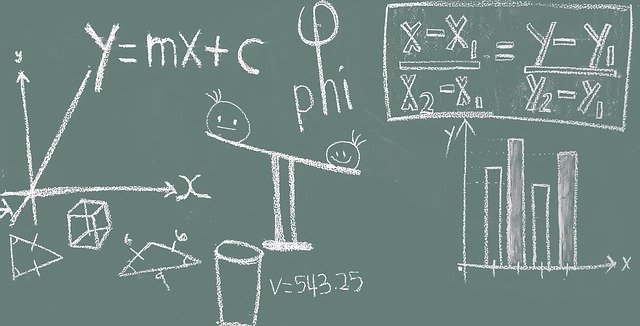Home » Blog » Amazing Maths Tricks To Wow Your Friends

Amazing Maths Tricks To Wow Your FriendsLike magic tricks, math tricks are also very exciting and interesting. But here is why maths tricks are better than magic; besides impressing your friends, you learn more about numbers and how they work. Tricks such as those we have listed below are maths made easy for beginners to understand the beauty of numbers.

4 Maths Tricks To Impress Your Friends

(A) Math Trick with the answer always equaling 5:1. Think of a number from 1 to 9. 2. Add 10 to it. 3. Add the original number again. 4. Divide the sum by 2.5. Subtract your original number. 6. Your answer equals 5!

(B) Math Trick Involving The Same Triple Digit 1. Think of a three digits number with the same digit throughout (for example, 888). 2. Add up the numbers. 3. Divide the original number with the added-up number. 4. The answer always equals 37!

(C) Multiplying Even numbers by 6: Do you know when you multiply single even numbers by 6, the first number of the answer in tens is actually half of the digit multiplied by six. The number attached to it is the exact number multiplied by 6!

(D) The Eleventh Rule Magic Trick: The eleventh rule works in two ways.

First: 1. Think of two digits numbers that add up to numbers less than 10 (e.g. 52).

3. Place the answer in between the two-digit number (572).

4. Divide the three digits numbers by 11.5. The answer equals the original two-digit number!

Secondly: 1. Think of two-digit numbers that add up to numbers in ten (e.g. 67).